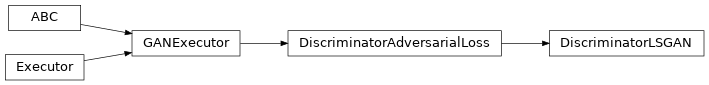# DiscriminatorLSGAN¶

Inheritance Diagramclass ashpy.losses.gan.DiscriminatorLSGAN[source]

Least square Loss for discriminator.

Reference: Least Squares Generative Adversarial Networks 1 .

Basically the Mean Squared Error between the discriminator output when evaluated in fake samples and 0 and the discriminator output when evaluated in real samples and 1: For the unconditioned case this is:

$L_{D} = \frac{1}{2} E[(D(x) - 1)^2 + (0 - D(G(z))^2]$

where x are real samples and z is the latent vector.

For the conditioned case this is:

$L_{D} = \frac{1}{2} E[(D(x, c) - 1)^2 + (0 - D(G(c), c)^2]$

where c is the condition and x are real samples.

1

Least Squares Generative Adversarial Networks https://arxiv.org/abs/1611.04076

Methods

 Initialize loss.

Attributes

 fn Return the Keras loss function to execute. global_batch_size Global batch size comprises the batch size for each cpu. weight Return the loss weight.
__init__()[source]

Initialize loss.

Return type

None